The Elegance of Exponents

INTRODUCTION

An exponent is generally expressed as follows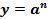In simple parlance we say that the original value of a “base” is operated upon by an “exponent” transforming it into some other value.

In subsequent sections, we will restrict variables to the sets described in the “APPENDIX: Infinite Number Sets”.  Initially we require that our base “a” is a real positive number.   This can be expressed formally for our “base” as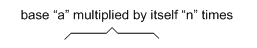INTEGER EXPONENTS

Early in the development of mathematics, exponents were invented as elegant shorthand for what would otherwise be a cumbersome series of repeated multiplications.   In the simplest case, we assume that our exponents, “m” and “n” are restricted to the positive integers so that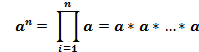So that the addition of a minus sign means thatUsing integers, an exponent indicates the number of times a base is multiplied by itself.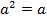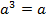* a* a * a

Then the expression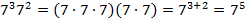is shorthand meaning the base “a” is to be multiplied by itself “n” times.  This leads us to two important relations.  The first case is when two terms with the same base, “a”, but different exponents are multiplied.  In this case, the exponents are added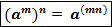The second case is when the quantity of a base with an exponent has a second exponent.  In this case, the exponents are multiplied.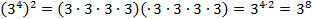and also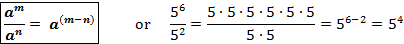As for example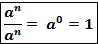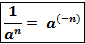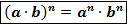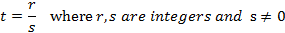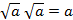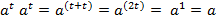If on the other hand we were to divide bases to various powers we might have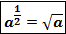Or for special cases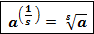For example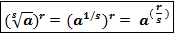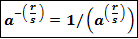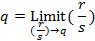RATIONAL NUMBER EXPONENTS

Rational numbers are those which can be expressed as a fraction with an integer for the numerator (number on top) divided by a non-zero integer for the denominator (number on the bottom.  If “r” and “s” are integers and s is not zero, we can express this asThen the fraction “t” is called a “rational” number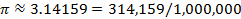An advantage of rational numbers is that we can use an exponent to represent a square root or indeed any arbitrary root.    Recall that the square root of “a”, is that number which when multiplied by itself gives a.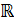But since exponents add for multiplications with the same base “a”, we can writeSolving for “t” we have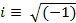So thatWe might then write, for exampleWe can generalize this result to note that a1/s is simply the “s-th” root of “a”, or the number which when multiplied by itself “s” times gives “a”.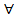Or more simply we simply have the “s-th” root of “a”, as follows:Continuing the analogy, we can use arbitrary fractions for the exponent, as given below: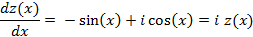or that a( r/s ) is the “s-th” root of the base “a” multiplied by itself “r” times.

And as before if the rational fraction for the exponent is negative, we can write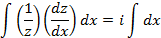IRRATIONAL NUMBER EXPONENTS

So far we have considered rational fractions, t = (r/s), where r and s are integers and s is not zero.  There are many other numbers, however, which cannot be expressed as any rational fraction and are therefore called “irrational.”

The most famous irrational number is the square root of two.  Sometime around the 6th century B.C. the Pythagorean Greeks discovered that any rational number fraction which might be equal to the square root of two had to have a numerator and a denominator both of which were infinitely divisible by two.  Since no integer has these properties, the square root of two cannot be expressed as a rational fraction.

Please note that all rational numbers, or fractions, when expressed as decimal numbers eventually have strings of digits that repeat forever.  Irrational numbers never have repeating sequences of digits.

Nevertheless for any irrational number, we can always find some rational fraction which approximates it to any degree of precision for which we have the patience.  That is to say that for any irrational number “q”, we can approximate it in the limit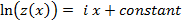Then as consequence, we can also write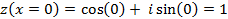One way to approximate “q” is to write it as a decimal for as many digits as are desired; and then substitute an integer divided by some power of ten.

For instance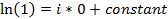REAL NUMBER EXPONENTS

The rational fractions and irrational numbers together comprise the number set called the “real” numbers,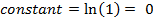.  And we have previously described exponents for both of these subsets which together constitute the entirety of the set

IMAGINARY NUMBER EXPONENTS

An interesting property of any real number, whether positive or negative is that if you multiply that number by itself, the result is always positive.  This means there is no “real” number that could be the square root of any negative “real” number.  This is a direct consequence of the fundamental assumption of algebra called the “Distributive Law” or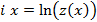For a variety of calculations which are essential to the development of modern technology, we need the square roots of negative numbers.  The solution was to invent a non-physical quantity called “i” which is defined as the square root of minus one.The set of all imaginary numbers,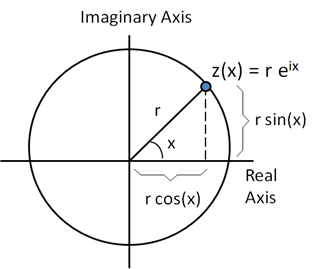, is simply every real number, “x”, multiplied by the weird constant “i”, as follows:=where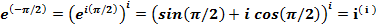simply means “for all”.   Around 1740 A.D., Swiss mathematician Euler discovered an elegant formula describing imaginary exponents.  The starting point is to define a function using trigonometric functions and the constant “i” as follows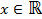Taking the derivative with respect to x, we get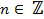Rearranging terms and integrating both sides with respect to “x” yields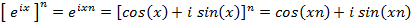The result of the term on the left hand side is the natural logarithm, ln(z), to base “e” which is a constant approximately equal to 2.718281828…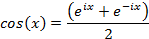But since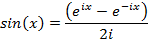Then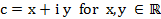or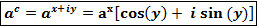The result is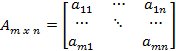And finally, we note the imaginary exponent has real and imaginary parts, as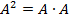This can be visualized as follows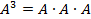Values along the “imaginary” axis are simply real numbers multiplied by “i”.  The angle x is expressed in radians.  Every point in the “complex” plane is thus some value of r*z(x) which has a real and imaginary part.    If r = 1, then every possible result for “ eix “ lies on the circumference of the corresponding circle.

Some bizarre examples relating fundamental numbers of nature include the following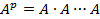What exactly is the significance of these relations is still to be determined.

Connecting imaginary numbers with trigonometry is de Moivre’s formula whenand, then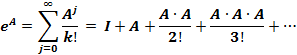Likewise we can write the trigonometric functions as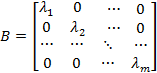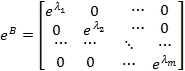COMPLEX NUMBER EXPONENTS

A “complex” number is defined as simply the sum of a real number and an imaginary number so for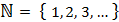then simplyEXPONENTS USING MATRICES

Matrices can also be raised to powers.  For instance we might have an arbitrary matrix “A” with “m” rows and “n” columns, then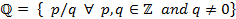If this matrix is square, “Am x m“, only then we can define a Natural number exponent “p” as follows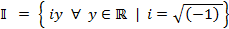or in general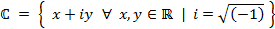where the matrix “A” is simply multiplied by itself “p” times.  And for the special casewhere the “Identity” matrix “I” has ones along the diagonal but zeros everywhere else.  This is not to be confused with the “Zero” matrix, Z”, which has zeros everywhere as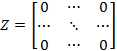Also note that the inverse matrix “A-1“ has a specifically different meaning than we might expect as followsBut a matrix can also be used as an exponent (so that “eA “ is itself a matrix) as followsNote that in the special case where the matrix has only diagonal non-zero elements asso thatAPPENDIX: Infinite Number Sets

Please note that we will use the following symbols to represent different well-known infinite number sets.- Natural or counting numbers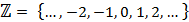- Integers- Rational numbers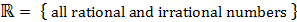- Real numbers- Imaginary numbers- Complex numbers

where “” simply means “for all”, “” means ”is a member of the set”, and “|” means “such that”.